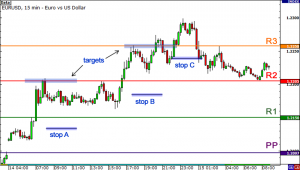# Pivot Points Binary StrategyBinary Options trading as well as Forex trading requires determination of entry levels using various reference points. But, most of the newbie binary traders fail to recognize the risk defining point as they rely highly on different technical indicators. Unprecedented risks can definitely be problematic for you but if you calculate your risks, your binary options strategy will certainly become more useful.

In this regard, the Pivot Point and its derivatives is a tool with the main purpose of providing defined support and resistance level while  minimizing the risk. As a matter of fact, good old technical tools are proven to work better in best binary options strategies in combination with Pivot Points rather than alone. Binary traders can use Pivot points very effectively and profitably. Read the following article to understand how.

## Best Binary Options Strategy: Pivot Points

The Pivot Points were originally developed for traders that use future trades and equity as their main instruments. Since the size of binary options trading market is large, it has a strong protection from possible market manipulations, the levels provided by pivot points used in best binary strategies actually work perfect in this market. In simple words, the Binary Options market follows resistance levels and support levels as well as other technical principals better most of the markets that are not as profitable.

## Calculating Pivots for Binary Trading Strategy

At any point of the day, you can calculate Pivot Points for binary instruments. The current trading day’s prices are calculated using the prices of previous day.

The general formula for calculating Pivot Points is:
Pivot Point for Current = {High (previous) + Low (previous) + Close (previous)}/3

Once you have calculated the pivot points, you can calculate the support and resistance levels for the trading day using them.

## Benefit of Using Binary Pivot Points

In order to get better idea of why pivot points are useful binary options strategy, take currency pair EUR/USD and create a statistical table showing for how apart each low and high has been from each resistance and support level.

Calculate yourself:

• Carry out a detailed analyses by estimating total pivot points as well as resistance and support levels for total number of days under consideration.
• Take the real lowest point of a trading day and subtract support point points from it. (low-S)
• Similarly, subtract pivot points for resistance from real high. (High-R)
• Find the mean Finally of each difference.

To make Pivot Points as Best binary Options Strategy more understandable, take the example of how Euros performance since its inception on Jan 4, 1999.

• On average, the actual low is 1 pip below Support 1.
• On average, the actual high is 1 pip high below Resistance 1.

For second support and resistance level:

• On average, the actual low is 53 pips above Support 2
• On average, the actual high is 53 pips below Resistance 2

## Judging Binary Strategy Pivot Probabilities

The above statistics clearly indicates that traders can have decent gauge of actual levels of a trading day with the help of Pivot Points calculated for S1 and R1. Furthermore, the total number of trading days after the inception of Euro according to calculated days for which high was higher than each R1, R2 and R3 and low was lower than each S1, S2 and S3 are 2,026.

The above information is very useful for traders to trade successfully. As for example, according to  the data that is presented above , the currency pair has 44% chance to fall below S1. It indicates that probability is favoring you and you can place the trade with confidence. Also, this binary options strategy shows that the real probability of highs for trading day exceed R1 only for 42 times out of hundred.

Although, there can be sudden moves that are unpredictable and this is not certain to happen as they are only probabilities yet the knowledge of calculating pivot points can help you to place trade at right times. Pivot points provide you an opportunity to measure the resistance and support level beforehand quite confidently, help in placing orders by accurately identifying reference points and this is the real power of Pivot Points Binary Options strategy.

As Binary Options Hub suggests, this is definitely the best binary strategy for those of you, who can understand it. In case you didn’t quite get it, you can always try our other binary strategies!

### 1 thought on “Pivot Points Binary Strategy”

1.Interesting read, really find this strategy worth of trying and hope to make success with it